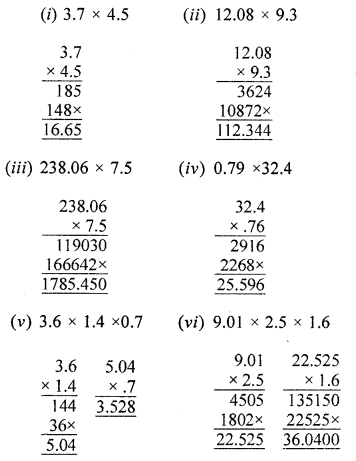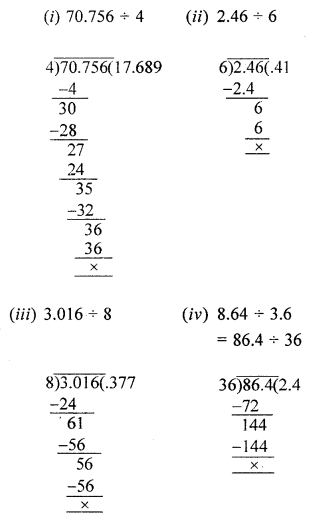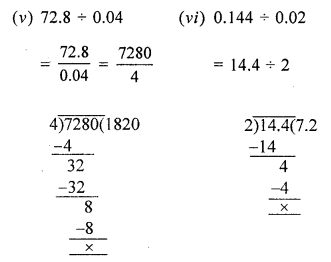## ML Aggarwal Class 6 Solutions for ICSE Maths Chapter 7 Decimals Ex 7.4

Question 1.
Evaluate the following:
(i) 3.7 × 4.5
(ii) 12.08 × 9.3
(iii) 238.06 × 7.5
(iv) 0.79 × 32.4
(v) 3.6 × 1.4 × 0.7
(vi) 9.01 × 2.5 × 1.6
Solution:Question 2.
Work out the following:
(i) 70.756 ÷ 4
(ii) 2.46 ÷ 6
(iii) 3.016 ÷ 8
(iv) 8.64 ÷ 3.6
(v) 72.8 ÷ 0.04
(vi) 0.144 ÷ 0.02
Solution:Question 3.
Multiply each of the following numbers by 10, 100 and 1000 orally ;
(i) 4.7
(ii) 3.45
(iii) 23.01
Solution:
(i) 4.7
4.7 × 10 = 47
4.7 × 100 = 470
4.7 × 1000 = 4700

(ii) 3.45
3.45 × 10 = 34.5
3.45 × 100 = 345
3.45 × 1000 = 3450

(iii) 0.234
0. 234 × 10 = 2.34
0.234 × 100 = 23.4
0.234 × 1000 = 234

Question 4.
Divide each of the following numbers by 10, 100 and 1000 (orally):
(i) 4.7
(ii) 3.45
(iii) 23.01
Solution:
(i) 4.7
4.7 ÷ 10 = 0.47
4.7 ÷ 100 = 0.047
4.7 ÷ 1000 = 0.0047

(ii) 3.45
3.45 ÷ 10 = 0.345
3.45 ÷ 100 = 0.0345
3.45 ÷ 1000 = 0.00345

(iii) 23.01
23.1 ÷ 10 = 2.301
23.1 ÷ 100 = 0.2301
23.1 ÷ 1000 = 0.02301